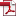• KSII Transactions on Internet and Information Systems
Monthly Online Journal (eISSN: 1976-7277)

# Design and Implementation of a Sequential Polynomial Basis Multiplier over GF(2m)

Vol. 11, No. 5, May 30, 201710.3837/tiis.2017.05.021, Download Paper (Free):#### Abstract

Finite field arithmetic over GF(2m) is used in a variety of applications such as cryptography, coding theory, computer algebra. It is mainly used in various cryptographic algorithms such as the Elliptic Curve Cryptography (ECC), Advanced Encryption Standard (AES), Twofish etc. The multiplication in a finite field is considered as highly complex and resource consuming operation in such applications. Many algorithms and architectures are proposed in the literature to obtain efficient multiplication operation in both hardware and software. In this paper, a modified serial multiplication algorithm with interleaved modular reduction is proposed, which allows for an efficient realization of a sequential polynomial basis multiplier. The proposed sequential multiplier supports multiplication of any two arbitrary finite field elements over GF(2m) for generic irreducible polynomials, therefore made versatile. Estimation of area and time complexities of the proposed sequential multiplier is performed and comparison with existing sequential multipliers is presented. The proposed sequential multiplier achieves 50% reduction in area-delay product over the best of existing sequential multipliers for m = 163, indicating an efficient design in terms of both area and delay. The Application Specific Integrated Circuit (ASIC) and the Field Programmable Gate Array (FPGA) implementation results indicate a significantly less power-delay and area-delay products of the proposed sequential multiplier over existing multipliers.